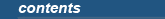Home |  JournalSeek |  SoftwareSeek |  GenomeSeek |  Expression |  Developer |  TakeOnItOperator overloading allows programmers to build types which feel as natural to use as simple types (int, long, etc.). C# implements a stricter version of operator overloading in C++, but it allows classes such as the quintessential example of operator-overloading, the complex number class, to work well.

In C#, the == operator is a non-virtual (operators can't be virtual) method of the object class which compares by reference. When you build a class, you may define your own == operator. If you are using your class with collections, then you should implement the IComparable interface. This interface has one method to implement, called the CompareTo (object) method, which should return positive, negative, or 0 if "this" is greater, less than, or the same value as the object. You may choose to define <, <=, >=, > methods if you want users of your class to have a nicer syntax. The numeric types (int, long, etc) implement the IComparable interface.

Here's a simple example of how to deal with equality and comparisons:

```public class Score : IComparable
{
int value;

public Score (int score) {
value = score;
}

public static bool operator == (Score x, Score y) {
return x.value == y.value;
}

public static bool operator != (Score x, Score y) {
return x.value != y.value;
}

public int CompareTo (object o) {
return value - ((Score)o).value;
}
}

Score a = new Score (5);
Score b = new Score (5);
Object c = a;
Object d = b;
```

To compare a and b by reference:

`System.Console.WriteLine ((object)a == (object)b; // false `

To compare a and b by value:

`System.Console.WriteLine (a == b); // true`

To compare c and d by reference:

`System.Console.WriteLine (c == d); // false`

To compare c and d by value:

`System.Console.WriteLine (((IComparable)c).CompareTo (d) == 0); // true`

You could also add the <, <=, >=, > operators to the score class. C# ensures at compile time that operators which are logically paired (!= and ==, > and <, >= and <=), must both be defined.

 C# 3.0 in a Nutshell (April 2007) - by Joseph Albahari and Ben Albahari.